As mentioned in my article about Monte Carlo estimate of (one-dimensional) integrals, one of the advantages of Monte Carlo integration is that you can perform multivariate integrals on complicated regions. This article demonstrates how to use SAS to obtain a Monte Carlo estimate of a double integral over rectangular and non-rectangular regions. Be aware that a Monte Carlo estimate is often less precise than a numerical integration of the iterated integral.

### Monte Carlo estimate of multivariate integrals

Multivariate integrals are notoriously difficult to solve. But if you use Monte Carlo methods, higher-dimensional integrals are only marginally more difficult than one-dimensional integrals. For simplicity, suppose we are interested in the double integral $\int_D f(x,y) \,dx\,dy$, where D is a region in the plane. The basic steps for estimating a multivariate integral are as follows:

1. Generate a large random sample of points from the uniform distribution on the domain, D.
2. Evaluate f at each point (x,y) in the random sample. Let W = f(X,Y) be the vector of values.
3. Compute Area(D)*mean(W), where Area(D) is the area of D and mean(W) is the arithmetic average of W. If you know the exact area of D, use it. If D is a very complicated shape, you can estimate the area of D by using the Monte Carlo methods in this article.

The quantity Area(D)*mean(W) is a Monte Carlo estimate of $\int_D f(x,y) \,dx\,dy$. The estimate depends on the size of random sample (larger samples tend to give better estimates) and the particular random variates in the sample.

### Monte Carlo estimates of double integrals on rectangular regions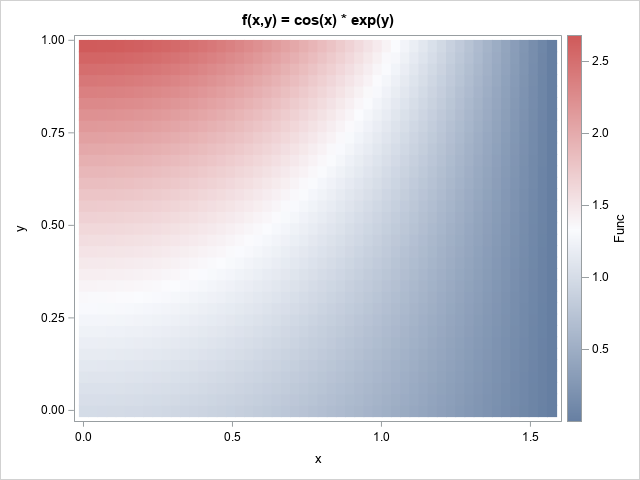Let's start with the simplest case, which is when the domain of integration, D, is a rectangular region. This section estimates the double integral of f(x,y) = cos(x)*exp(y) over the region D = [0,π/2] x [0,1]. That is, we want to estimate the integral
$\int\nolimits_0^{\pi/2}\int\nolimits_0^1 \cos(x)\exp(y)\,dy\,dx = e - 1 \approx 1.71828$
where e is the base of the natural logarithm. For this problem, the area of D is (b-a)*(d-c) = π/2. The graph at the right shows a heat map of the function on the rectangular domain.

The following SAS/IML program defines the integrand and the domain of integration. The program generates 5E6 uniform random variates on the interval [a,b]=[0,π/2] and on the interval [c,d] = [0,1]. The (x,y) pairs are evaluated, and the vector W holds the result. The Monte Carlo estimate is the area of the rectangular domain times the mean of W:

/* Monte Carlo approximation of a double integral over a rectangular region */ proc iml; /* define integrand: f(x,y) = cos(x)*exp(y) */ start func(x); return cos(x[,1]) # exp(x[,2]); finish;   /* Domain of integration: D = [a,b] x [c,d] */ pi = constant('pi'); a = 0; b = pi/2; /* 0 < x < pi/2 */ c = 0; d = 1; /* 0 < y < 1 */   call randseed(1234); N = 5E6;   X = j(N,2); /* X ~ U(D) */ z = j(N,1); call randgen(z, "uniform", a, b); /* z ~ U(a,b) */ X[,1] = z; call randgen(z, "uniform", c, d); /* z ~ U(c,d) */ X[,2] = z;   W = func(X); /* W = f(X1,X2) */ Area = (b-a)*(d-c); /* area of rectangular region */ MCEst = Area * mean(W); /* MC estimate of double integral */   /* the double integral is separable; solve exactly */ Exact = (sin(b)-sin(a))*(exp(d)-exp(c)); Diff = Exact - MCEst; print Exact MCEst Diff;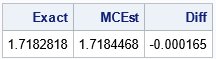The output shows that the Monte Carlo estimate is a good approximation to the exact value of the double integral. For this random sample, the estimate is within 0.0002 units of the true value.

### Monte Carlo estimates of double integrals on non-rectangular regions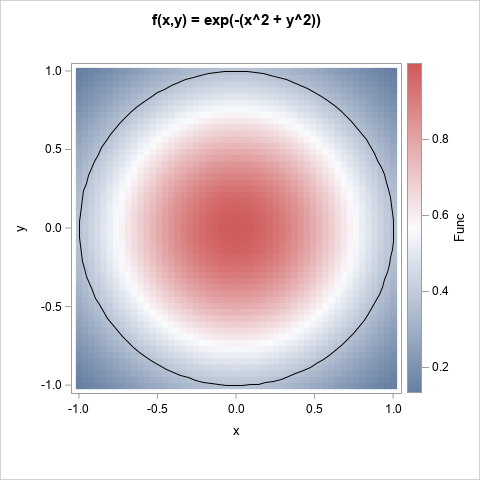It is only slightly more difficult to estimate a double integral on a non-rectangular domain, D. It is helpful to split the problem into two subproblems: (1) generate point uniformly in D, and (2) estimate the integral on D.

The next two sections show how to estimate the integral of f(x,y) = exp(-(x2 + y2)) over the circle of unit radius centered at the origin: D = {(x,y) | x2 + y2 ≤ 1}. The graph at the right shows a heat map of the function on a square. The domain of integration is the unit disk.

By using polar coordinates, you can solve this integral exactly. The double integral has the value 2π*(e – 1)/(2 e) ≈ 1.9858653, where e is the base of the natural logarithm.

### Generate point uniformly in a non-rectangular planar region

For certain shapes, you can directly generate a random sample of uniformly distributed points:

In general, for any planar region, you can use the acceptance-rejection technique. I previously showed an example of using Monte Carlo integration to estimate π by estimating the area of a circle.

Because the acceptance-rejection technique is the most general, let's use it to generate a random set of points in the unit disk, D. The steps are as follows:
1. Construct a bounding rectangle for the region. In this example, you can use R = [-1,1] x [-1,1].
2. Generate random points uniformly at random in the rectangle.
3. Keep the points that are in the domain, D. Discard the others.

If you know the area of D, you can use a useful trick to choose the sample size. When you use an acceptance-rejection technique, you do not know in advance how many points will end up in D. However, you can estimate the number as ND ≈ NR*Area(D)/Area(R), where NR is the sample size in R. If you know the area of D, you can invert this formula. If you want approximately ND points to be in D, generate ND*Area(R)/Area(D) points in R. For example, suppose you want ND=5E6 points in the unit disk, D. We can choose NR ≥ ND*4/π, as in the following SAS/IML program, which generates random points in the unit disk:

proc iml; /* (1) Generate approx N_D points in U(D), where D is the unit disk D = { (x,y) | x**2 + y**2 <= 1 } */ N_D = 5E6; /* we want this many points in D */ a = -1; b = 1; /* Bounding rectangle, R: */ c = -1; d = 1; /* R = [a,b] x [c,d] */   /* generate points inside R. Generate enough points (N_R) in R so that approximately N_D are actually in D */ pi = constant('pi'); area_Rect = (b-a)*(d-c); area_D = pi; N_R = ceil(N_D * area_Rect / area_D); /* estimate how many points in R we'll need */   call randseed(1234); X = j(N_R,2); z = j(N_R,1); call randgen(z, "uniform", a, b); X[,1] = z; call randgen(z, "uniform", c, d); X[,2] = z;   /* which points in the bounding rectangle are in D? */ b = (X[,##] <= 1); /* x^2+y^2 <= 1 */ X = X[ loc(b),]; /* these points are in D */ print N_D[L="Target N_D" F=comma10.] (nrow(X))[L="Actual N_D" F=comma10.];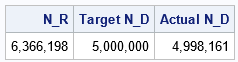The table shows the result. The program generated 6.3 million points in the rectangle, R. Of these, 4.998 million were in the unit disk, D. This is very close to the desired number of points in D, which was 5 million.

### Monte Carlo estimate of a an integral over a planar region

Each row of the matrix, X, contains a point in the disk, D. The points are a random sample from the uniform distribution on D. Therefore, you can estimate the integral by calculating the mean value of the function on these points:

/* (2) Monte Carlo approximation of a double integral over a non-rectangular domain. Estimate integral of f(x,y) = exp(-(x**2 + y**2)) over the disk D = { (x,y) | x**2 + y**2 <= 1 } */ /* integrand: f(x,y) = exp(-(x**2 + y**2)) */ start func(x); r2 = x[,1]##2 + x[,2]##2; return exp(-r2); finish;   W = func(X); MCEst = Area_D * mean(W);   /* compare the estimate to the exact value of the integral */ e = constant('e'); Exact = 2*pi*(e-1)/(2*e); Diff = Exact - MCEst; print Exact MCEst Diff;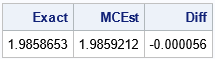The computation shows that a Monte Carlo estimate of the integral over the unit disk is very close to the exact value. For this random sample, the estimate is within 0.00006 units of the true value.

### Summary

This article shows how to use SAS to compute Monte Carlo estimates of double integrals on a planar region. The first example shows a Monte Carlo integration over a rectangular domain. The second example shows a Monte Carlo integration over a non-rectangular domain. For a non-rectangular domain, the integration requires two steps: first, use an acceptance-rejection technique to generate points uniformly at random in the domain; then use Monte Carlo to estimate the integral. The technique in this article generalizes to integration on higher-dimensional domains.

The post Double integrals by using Monte Carlo methods appeared first on The DO Loop.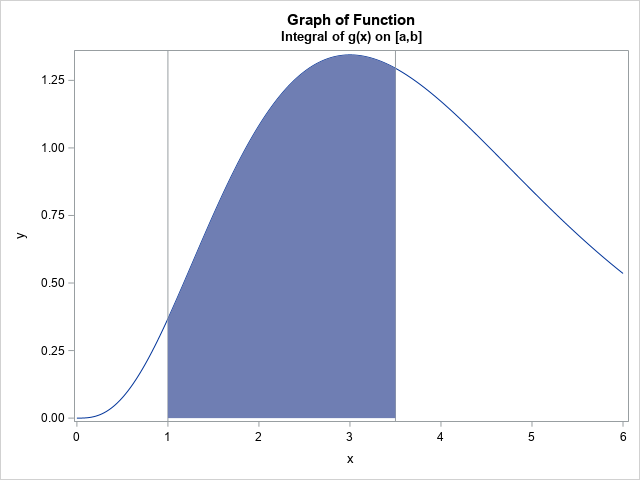A previous article shows how to use Monte Carlo simulation to estimate a one-dimensional integral on a finite interval. A larger random sample will (on average) result in an estimate that is closer to the true value of the integral than a smaller sample. This article shows how you can determine a sample size so that the Monte Carlo estimate is within a specified distance from the true value, with high probability.

### Ensure the estimate is (probably) close to the true value

As shown in the previous article, a Monte Carlo estimate of an integral of g(x) on the interval (a,b) requires three steps:

1. Generate a uniform random sample of size n on (a,b). Let X be the vector of n uniform random variates.
2. Use the function g to evaluate each point in the sample. Let Y = g(X) be the vector of transformed variates.
3. An estimate of the integral $\int\nolimits_{a}^{b} g(x) \, dx$ is (b - a)*mean(Y), where mean(Y) is the arithmetic average of Y, as shown in the previous article.

The goal of this article is to choose n large enough so that, with probability at least β, the Monte Carlo estimate of the integral is within δ of the true value. The mathematical derivation is at the end of this article. The result (Neal, 1983, p. 257) is that you should choose
$n > \left( \Phi^{-1}\left( \frac{\beta+1}{2} \right) \frac{(b-a)s_{Y}}{\delta} \right)^2$
where $\Phi^{-1}$ is the quantile function of the standard normal distribution, and $s_{Y}$ is an estimate of the standard deviation of Y.

### Applying the formula to a Monte Carlo estimate of an integral

Let's apply the formula to see how large a sample size we need to estimate the integral $\int\nolimits_{a}^{b} g(x) \, dx$ to three decimal places (δ=5E-4) for the function g(x) = xα-1 exp(-x), where α=4 and the interval of integration is (1, 3.5). The function is shown at the top of this article.

The estimate requires knowing an estimate for the standard deviation of Y=g(X), where X ~ U(a,b). You can use a small "pilot study" to obtain the standard deviation, as shown in the following SAS/IML program. You could also use the DATA step and PROC MEANS for this computation.

%let alpha = 4; /* shape parameter */ %let a = 1; /* lower limit of integration */ %let b = 3.5; /* upper limit of integration */   proc iml; /* define the integrand */ start Func(x) global(shape); return x##(shape - 1) # exp(-x); finish;   call randseed(1234); shape = &alpha; a = &a; b = &b;   /* Small "pilot study" to estimate s_Y = stddev(Y) */ N = 1e4; /* small sample */ X = j(N,1); call randgen(x, "Uniform", a, b); /* X ~ U(a,b) */ Y = func(X); /* Y = f(X) */ s = std(Y); /* estimate of std dev(Y) */

For this problem, the estimate of the standard deviation of Y is about 0.3. For β=0.95, the value of $\Phi^{-1}((\beta+1)/2) \approx 1.96$, but the following program implements the general formula for any probability, β:

/* find n so that MC est is within delta of true value with 95% prob */ beta = 0.95; delta = 5e-4; k = quantile("Normal", (beta+1)/2) * (b-a) * s; sqrtN = k / delta; roundN = round(sqrtN**2, 1000); /* round to nearest 1000 */ print beta delta roundN[F=comma10.];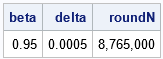With 95% probability, if you use a sample size of n=8,765,000, the Monte Carlo estimate will be within δ=0.0005 units of the true value of the integral. Wow! That's a larger value than I expected!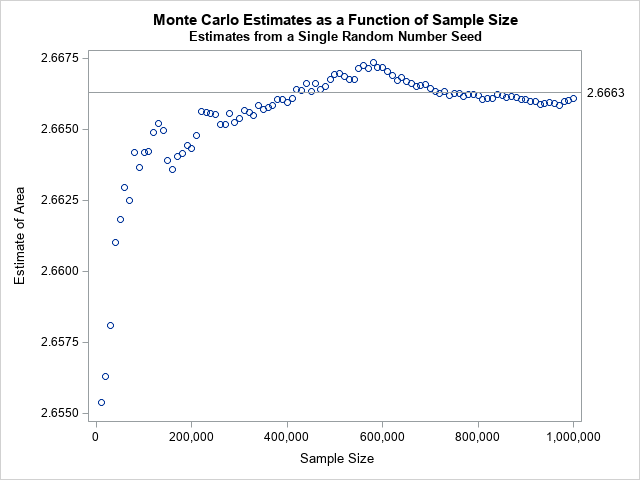I used n=1E6 in the previous article and reported that the difference between the Monte Carlo approximation and the true value of the integral was less than 0.0002. So it is possible to be close to the true value by using a smaller value of n. In fact, the graph to the right (from the previous article) shows that for n=400,000 and n=750,000, the Monte Carlo estimate is very close for the specific random number seed that I used. But the formula in this section provides the sample size that you need to (probably!) be within ±0.0005 REGARDLESS of the random number seed.

### The distribution of Monte Carlo estimates

I like to use SAS to check my math. The Monte Carlo estimate of the integral of g will vary according to the random sample. The math in the previous section states that if you generate k random samples of size n=8,765,000, that (on average) about 0.95*k of the sample will be within δ=0.0005 units of the true value of the integral, which is 2.666275. The following SAS/IML program generates k=200 random samples of size n and k estimates of the integral. We expect about 190 estimates to be within δ units of the true value and only about 10 to be farther away:

N = roundN; X = j(N,1);   k = 200; Est = j(k,1); do i = 1 to k; call randgen(X, "Uniform", a, b); /* x[i] ~ U(a,b) */ Y = func(X); /* Y = f(X) */ f_avg = mean(Y); /* estimate E(Y) */ Est[i] = (b-a)*f_avg; /* estimate integral */ end;   call quad(Exact, "Func", a||b); /* find the "exact" value of the integral */ Diff = Est - Exact; title "Difference Between Monte Carlo Estimate and True Value"; title2 "k=200 Estimates; n=8,765,000"; call Histogram(Diff) other="refline -0.0005 0.0005/axis=x;";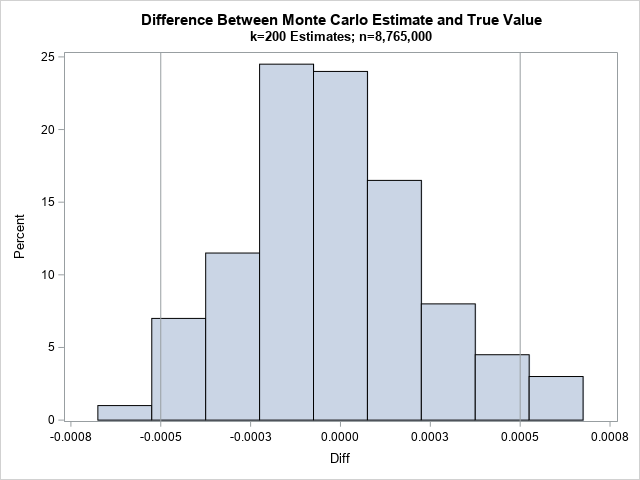The histogram shows the difference between the exact integral and the estimates. The vertical reference lines are at ±0.0005. As predicted, most of the estimates are less than 0.0005 units from the exact value. What percentage? Let's find out:

/* how many estimates are within and NOT within delta? */ Close = ncol(loc(abs(Diff)<=delta)); /* number that are closer than delta to true value */ NotClose = ncol(loc(abs(Diff)> delta)); /* number that are farther than delta */ PctClose = Close / k; /* percent close to true value */ PctNotClose = NotClose / k; /* percent not close */ print k Close NotClose PctClose PctNotClose;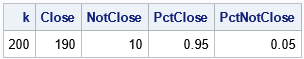For this set of 200 random samples, exactly 95% of the estimates were accurate to within 0.0005. Usually, a simulation of 200 estimates would show that between 93% and 97% of the estimates are close. In this case, the answer was exactly 95%, but don't expect that to happen always!

### Summary

This article shows how you can use elementary statistics to find a sample size that is large enough so that a Monte Carlo estimate is (probably!) within a certain distance of the exact value. The article was inspired by an article by Neal, D. (1983). The mathematical derivation of the result is provided in the next section.

### Derivation of the sample size formula

This section derives the formula for choosing the sample size, n. Because the Monte Carlo estimate is a mean, you can use elementary probability theory to find n. Let {x1, x2, ..., xn} be random variates on (a, b). Let Y be the vector {g(x1), g(x2), ..., g(xn)}. Let $m_{n} = \frac{1}{n} \sum\nolimits_{i = 1}^{n} g(x_{i})$ be the mean of Y and let $\mu = \frac{1}{b-a} \int\nolimits_{a}^{b} g(x) \, dx$ be the average value of g on the interval (a,b). We know that $m_n \to \mu$ as $n \to \infty$. For any probability 0 < β < 1, we want to find n large enough so that
$P\left( | m_n - \mu | < \frac{\delta}{(b-a)} \right) \geq \beta$
From the central limit theorem, we can substitute the standard normal random variable, Z, inside the parentheses by dividing by the standard error of Y, which is σY/sqrt(n):
$P\left( | Z | < \frac{\delta \sqrt{n}}{(b-a)\sigma_{Y}} \right) \geq \beta$
Equivalently,
$2 P\left( Z < \frac{\delta \sqrt{n}}{(b-a)\sigma_{Y}} \right) - 1 \geq \beta$

Solving the equation for sqrt(n) gives
$\sqrt{n} > \Phi^{-1}\left( \frac{\beta+1}{2} \right) \frac{(b-a)\sigma_{Y}}{\delta}$
Squaring both sides leads to the desired bound on n. Because we do not know the true standard deviation of Y, we substitute a sample statistic, sY.

The post Sample size for the Monte Carlo estimate of an integral appeared first on The DO Loop.Numerical integration is important in many areas of applied mathematics and statistics. For one-dimensional integrals on the interval (a, b), SAS software provides two important tools for numerical integration:

This article shows a third method to estimate an integral in SAS: Monte Carlo simulation.

### How to use Monte Carlo simulation to estimate an integral

I previously showed an example of using Monte Carlo simulation to estimate the value of pi (π) by using the "average value method." This section presents the mathematics behind the Monte Carlo estimate of the integral.

The Monte Carlo technique takes advantage of a theorem in probability that is whimsically called the Law of the Unconscious Statistician. The theorem is basically the chain rule for integrals. If X is a continuous random variable and Y = g(X) is a random variable that is created by a continuous transformation (g) of X, then the expected value of Y is given by the following convolution:
$E[Y] = E[g(X)] = \int g(x) f_{X}(x) \,dx$
where $f_{X}$ is the probability density function for X.

To apply the theorem, choose X to be a uniform random variable on the interval (a,b). The density function of X is therefore $f_{X}(x) = 1/(b-a)$ if x is in (a,b) and 0 otherwise. Rearranging the equation gives
$\int_{a}^{b} g(x) \,dx = (b - a) \cdot E[g(X)]$

Consequently, to estimate the integral of a continuous function g on the interval (a,b), you need to estimate the expected value E[g(X)], where X ~ U(a,b). To do this, generate a uniform random sample in (a,b), evaluate g on each point in the sample, and take the arithmetic mean of those values. In symbols,
$\int_{a}^{b} g(x) \,dx \approx (b - a) \cdot \frac{1}{n} \sum\nolimits_{i = 1}^{n} g(x_{i})$
where the $x_i$ are indepenent random uniform variates on (a,b).

### Estimate an integral in SAS by using Monte Carlo simulation

Suppose you want to estimate the integral of $g(x) = x^{\alpha - 1} \exp(-x)$ on the interval (a,b) = (1, 3.5). The figure at the top of this article shows the graph of g for α=4 and the area under the curve on the interval (1, 3.5).

As mentioned earlier, an accurate way to numerically integrate the function is to use the QUAD subroutine in SAS/IML, as follows:

%let alpha = 4; /* shape parameter */ %let a = 1; /* lower limit of integration */ %let b = 3.5; /* upper limit of integration */   proc iml; /* define the integrand */ start Func(x) global(shape); return x##(shape - 1) # exp(-x); finish;   /* call the QUAD subroutine to perform numerical integration */ shape = &alpha; a= &a; b = &b; call quad(AreaQuad, "Func", a||b); print AreaQuad;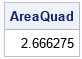The QUAD subroutine approximates the area under the curve as 2.6663. This is a very accurate result. When you compute a Monte Carlo estimate, the estimate will depend on the size of the random sample that you use and the random number seed. The following SAS/IML program samples one million random variates from the uniform distribution on [1, 3.5]. The vector Y contains the transformed points (Y=g(X)). The call to the MEAN function estimates the expected value of g(x) when 1 < x < 3.5. If you multiply the mean by (3.5 - 1) = 2.5, you obtain an estimate of the integral, as follows:

/* Monte Carlo estimation of the integral */ call randseed(1234); N = 1e6; /* sample size for MC estimate */ X = j(N,1); /* allocate vector of size N */ call randgen(X, "Uniform", a, b); /* x[i] ~ U(a,b) */ Y = func(X); /* Y = f(X) */ f_avg = mean(Y); /* estimate E(Y) */ AreaMC = (b-a)*f_avg; /* estimate integral on (a,b) */ Diff = abs(AreaMC - AreaQuad); /* how close is the estimate to the true value? */ print AreaQuad AreaMC Diff;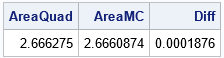### Advantages and drawbacks of a Monte Carlo estimate

The main advantage of a Monte Carlo estimate is its simplicity: sample, evaluate, average. The same technique works for any function over any finite interval of integration. Although I do not demonstrate it here, a second advantage is that you can extend the idea to estimate higher-dimensional integrals.

The main disadvantage is that you do not obtain a definitive answer. You obtain a statistical estimate that is based on a random sample. I won't repeat the arguments from my article about Monte Carlo estimates, but be aware of the following facts about a Monte Carlo estimate of an integral:

• Unless you use a huge sample size, the Monte Carlo estimate is usually less accurate than numerical integration.
• A Monte Carlo estimate (like all statistics) has a distribution. If you change the random number seed or you change the algorithm that you use to generate random uniform variates, you will get a different estimate. Thus, you cannot talk about the Monte Carlo estimate, but only about a Monte Carlo estimate. The best you can claim is that the true value of the integral is within a certain distance from your estimate (with a specified probability).

### How does the accuracy of the estimate depend on the sample size?

You can extend the previous program to demonstrate the accuracy of the Monte Carlo estimate as a function of the sample size. How would the estimate change if you use only 50,000 or 100,000 random variates? The following program creates a graph the shows the estimate of the integral when you use only the first k elements in the sequence of random variates:

/* how does the estimate depend on the sample size? */ size = do(1E4, N, 1E4); MCest = j(1,ncol(size),.); do i = 1 to ncol(size); Z = X[1:size[i]]; /* sample size k=size[i] */ Y = func(Z); /* Y = f(Z) */ MCEst[i] = (b-a)*mean(Y); /* estimate integral */ end;   title "Monte Carlo Estimates as a Function of Sample Size"; stmt = "refline " + char(AreaQuad,6,4) + "/ axis=y label; format size comma10.;"; call scatter(size, MCEst) other=stmt label={"Sample Size" "Estimate of Area"};The markers in the scatter plot show the estimates for the integral when only the first k random variates are used. The horizontal line shows the exact value of the integral. For this random-number seed, the estimate stays is close to the exact value when the sample size is more than 400,000. If you use a different random number seed, you will get a different graph. However, this graph is fairly typical. The Monte Carlo estimates can either overestimate or underestimate the integral.

The takeaway lesson is that the estimate converges to the exact value, but not very quickly. From general theory, we know that the standard error of the mean of n numbers is SD(Y)/sqrt(n), where SD(Y) is the standard deviation of Y for Y=g(X). That means that a 95% confidence interval for the mean has a radius 1.96*SD(Y)/sqrt(n). You can use this fact to choose a sample size so that the Monte Carlo estimate is (probably) close to the exact value of the integral. I will discuss this fact in a second article.

### Summary

This article shows how to use Monte Carlo simulation to estimate a one-dimensional integral. Although Monte Carlo simulation is less accurate than other numerical integration methods, it is simple to implement and it readily generalizes to higher-dimensional integrals.

The post Estimate an integral by using Monte Carlo simulation appeared first on The DO Loop.This is my Pi Day post for 2021. Every year on March 14th (written 3/14 in the US), geeky mathematicians and their friends celebrate "all things pi-related" because 3.14 is the three-decimal approximation to pi. Most years I write about lower-case pi (π), which is the ratio of a circle's circumference to its diameter. But this year I thought it would be fun to write about upper-case pi ($\Pi$), which is the mathematical notation for a product of terms.

Just as $\Sigma$ is used to express a summation, so $\Pi$ is used to express a product. Did you know that there are infinite products that converge to pi? A famous one is the Wallis product, which converges to π/2:
$\pi / 2 = \prod_{n=1}^{\infty} \left( \frac{2n}{2n-1} \cdot \frac{2n}{2n+1} \right) = \left( \frac{2}{1}\cdot \frac{2}{3} \right) \cdot \left( \frac{4}{3}\cdot \frac{4}{5} \right) \cdot \left( \frac{6}{5}\cdot \frac{6}{7} \right) \cdot \left( \frac{8}{7}\cdot \frac{8}{9} \right) \cdot \;\cdots$

### Implement Wallis's formula for pi

Suppose you wanted to implement Wallis's formula on a computer. How would you do it? The simplest way is to literally translate the formula into a loop that multiplies the first N terms in the product. You can plot the partial products against the number of terms to see how quickly (or slowly!) the product converges, as shown in the following SAS DATA step:

ods graphics / reset; %let N = 1000; data WallisProd; prod = 1; do n = 1 to &N; term = (2*n/(2*n-1)) * (2*n/(2*n+1)); prod = prod * term; output; end; run;   title "Convergence of Wallis Product to pi/2"; proc sgplot data=WallisProd; where n >= 100; scatter x=n y=prod; refline &pi2 / axis=y noclip lineattrs=(color=red) label="pi/2"; xaxis grid; yaxis grid; run;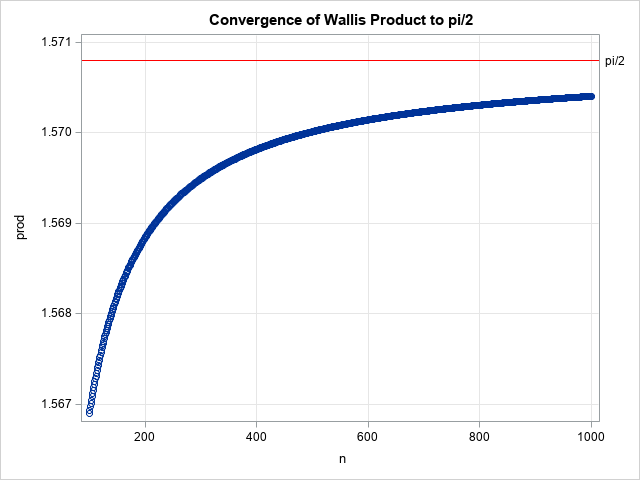The graph indicates that the convergence of the Wallis product is very slow. In fact, you can prove that the n_th term of the product has an asymptotic error of size π/(8n) as n → ∞. Because of this, the Wallis formula is not a good choice for computing the digits of pi. It takes about 400,000 terms to get six-digit accuracy!

But let's not focus on π, let's focus on the product, $\Pi$. Although a direct translation of the product formula is simple, it is not necessarily the best way to compute the product, computationally speaking. Pi Day seems like a great time to review an alternative computational method that is often useful when you compute with products.

### Products and log transformations in statistics

The Wallis product is well behaved because the individual terms are all close to 1. However, many products that you encounter in computational statistics are not so well behaved. For example, in statistical modeling, you encounter the likelihood function. Given a set of data (x1, x2,..., xn) and a probability density function (f) that you believe models the data, the likelihood of the data is the product $\prod_{i=1}^{n} f(x_{i})$. In this formulation, every term in the product is less than 1. If the data contain outliers, there might be individual probabilities that are tiny. Therefore, a naive computation of the product can result in a very small number. In fact, the computation might encounter an underflow error, especially for large data sets.

A computational technique that avoids this potential problem is to apply a log transformation, which converts the product into a summation of the log of the terms. After you have computed the sum, you exponentiate it to obtain the product. This technique requires that all terms in the product be positive, which is true in most applications.

In symbols, if you have a product of positive values, $y = \prod_{i} a_{i}$, you can log-transform it to $\log(y) = \sum_{i} \log(a_{i})$. The product is mathematically equivalent to $y = \exp\left( \sum_{i} \log(a_{i}) \right)$.

Let's apply the log transformation to the Wallis formula for π/2. The following SAS DATA step computes a sum of log-transformed terms. After each step, the EXP function gives the value of the product at that step.

data WallisSum; do n = 1 to &N; logterm = 2*log(2*n) - log(2*n-1) - log(2*n+1); /* log transform of terms */ logprod + logterm; /* summation */ prod = exp(logprod); /* exp(sum) */ output; end; run;

The graph of the partial products is the same graph as in the previous section. The numerical difference between the two computations is less than 3E-14. However, in general, it is usually safer to sum the log-transformed terms because the method avoids numerical overflow and underflow issues that are common when you compute a product in a naive way.

So, this Pi Day, don't just celebrate lower-case pi, π = 3.14159265.... Take a moment to think about upper-case pi ($\Pi$), which stands for a product of numbers. For many products, a numerically stable way to compute the product to log-transform the problem, compute a summation, and then exponentiate the sum. And that is a numerical technique that is worth celebrating, not just on Pi Day but every day!

The post Pi and products appeared first on The DO Loop.Finite-precision computations can be tricky. You might know, mathematically, that a certain result must be non-negative or must be within a certain interval. However, when you actually compute that result on a computer that uses finite-precision, you might observe that the value is slightly negative or slightly outside of the interval. This frequently happens when you are adding numbers that are not exactly representable in base 2. One of the most famous examples is the sum
0.1 + 0.1 + 0.1 ≠ 0.3 (finite precision),
which is shown to every student in Computer Science 101. Other examples include:

• If x is in the interval [0,1], then y = 1 - x10 is also in the interval [0,1]. That is true in exact precision, but not necessarily true in finite-precision computations when x is close to 1.
• Although sin2(t) + cos2(t) = 1 in exact arithmetic for all values of t, the equation might not be true in finite precision.

SAS programs that demonstrate the previous finite-precision computations are shown at the end of this article. Situations like these can cause problems when you want to use the result in a function that has a restricted domain. For example, the SQRT function cannot operate on negative numbers. For probability distributions, the quantile function cannot operate on numbers outside the interval [0,1].

This article discusses how to "trap" results that are invalid because they are outside of a known interval. You can use IF-THEN/ELSE logic to catch an invalid result, but SAS provides more compact syntax. This article discusses using the IFN function in Base SAS and using the "elementwise minimum" (<>) and "elementwise maximum" (><) operators in the SAS/IML language.

### Trap and map

I previously wrote about the "trap and cap" technique for handling functions like log(x). The idea is to "trap" invalid input values (x ≤ 0) and "cap" the output when you are trying to visualize the function. For the present article, the technique is "trap and map": you need to detect invalid computations and then map the result back into the interval that you know is mathematically correct. For example, if the argument is supposed to represent a probability in the interval [0,1], you must map negative numbers to 0 and map numbers greater than 1 to 1.

### How to use the IFN function in SAS

Clearly, you can "trap and map" by using IF-THEN/ELSE logic. For example, suppose you know that a variable, x, must be in the interval [0,1]. You can use the SAS DATA step to trap invalid values and map them into the interval, as follows:

/* trap invalid values of x and map them into [0,1]. Store result in y */ data Map2; input x @@; if x<0 then y = 0; else if x>1 then y = 1; else y = x; datalines; 1.05 1 0.9 0.5 0 -1e-16 -1.1 ;

This program traps the invalid values, but it requires six lines of IF-THEN/ELSE logic. A more compact syntax is to use the IFN function. The IFN function has three arguments:

• The first argument is a logical expression, such as x < 0.
• The second argument is the value to return when the logical expression is true.
• The third argument is the value to return when the logical expression is false.

For example, you can use the function call IFN(x<0, 0, x) to trap negative values of x and map those values to 0. Similarly, you can use the function call IFN(x>1, 1, x) to trap values of x that are greater than 1 and map those values to 1. To perform both of the trap-and-map operations on one line, you can nest the function calls so that the third argument to the IFN function is itself a function call, as follows:

data Map2; input x @@; y = ifn(x<0, 0, ifn(x>1, 1, x)); /* trap and map: force x into [0,1] */   /* or, for clarity, split into two simpler calls */ temp = ifn(x>1, 1, x); /* force x <= 1 */ z = ifn(x<0, 0, temp); /* force x >= 0 */ datalines; 1.05 1 0.9 0.5 0 -1e-16 -1.1 ;   proc print noobs; run;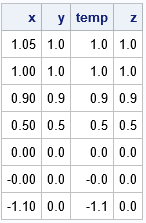The output shows that the program performed the trap-and-map operation correctly. All values of y are in the interval [0,1].

If you can't quite see how the logic works in the statement that nests the two IFN calls, you can split the logic into two steps as shown later in the program. The first IFN call traps any variables that are greater than 1 and maps them to 1. The second IFN call traps any variables that are less than 0 and maps them to 0. The z variable has the same values as the y variable.

### How to use the element min/max operators in SAS/IML

The SAS/IML language contains operators that perform similar computations. If x is a vector of numbers, then

• The expression (x <> 0) returns a vector that is the elementwise maximum between the elements of x and 0. In other words, any elements of x that are less than 0 get mapped to 0.
• The expression (x >< 1) returns a vector that is the elementwise minimum between the elements of x and 1. In other words, any elements of x that are greater than 1 get mapped to 1.
• You can combine the operators. The expression (x <> 0) >< 1 forces all elements of x into the interval [0,1]. Because the elementwise operators are commutative, you can also write the expression as 0 <> x >< 1.

These examples are shown in the following SAS/IML program:

proc iml; x = {1.05, 1, 0.9, 0.5, 0, -1e-16, -1.1}; y0 = (x <> 0); /* force x >= 0 */ y1 = (x >< 1); /* force x <= 1 */ y01 = (x <> 0) >< 1; /* force x into [0,1] */ print x y0 y1 y01;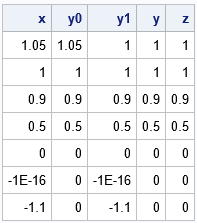### Summary

In summary, because of finite-precision computations (or just bad input data), it is sometimes necessary to trap invalid values and map them into an interval. Using IF-THEN/ELSE statements is clear and straightforward, but require multiple lines of code. You can perform the same logical trap-and-map calculations more compactly by using the IFN function in Base SAS or by using the elementwise minimum or maximum operators in SAS/IML.

### Appendix: Examples of floating-point computations to study

The following SAS DATA step programs show typical computations for which the floating-point computation can produce results that are different from the expected mathematical (full-precision) computation.

data FinitePrecision; z = 0.1 + 0.1 + 0.1; if z^=0.3 then put 'In finite precision: 0.1 + 0.1 + 0.1 ^= 0.3'; run;   data DomainError1; do t = -4 to 4 by 0.1; x = cos(t); y = sin(t); z = x**2 + y**2; /* always = 1, mathematically */ s = sqrt(1 - z); /* s = sqrt(0) */ output; end; run;   data DomainError2; do x = 0 to 1 by 0.01; z = 1 - x**10; /* always in [0,1], mathematically */ s = sqrt(z); output; end; run;Finding the root (or zero) of a nonlinear function is an important computational task. In the case of a one-variable function, you can use the SOLVE function in PROC FCMP to find roots of nonlinear functions in the DATA step. This article shows how to use the SOLVE function to find the roots of a user-defined function from the SAS DATA step.

### Some ways to find the root of a nonlinear function in SAS

I have previously blogged about several ways to find the roots of nonlinear equations in SAS, including:

The DATA step does not have a built-in root-finding function, but you can implement one by using PROC FCMP.

### PROC FCMP: Implement user-defined functions in SAS

PROC FCMP enables you to write user-defined functions that can be called from the DATA step and from SAS procedures that support DATA step programming statements (such as PROC NLIN, PROC NLMIXED, and PROC MCMC). To demonstrate finding the roots of a custom function, let's use the same function that I used to show how to find roots by using SAS/IML. The following statements use PROC FCMP to define a function (FUNC1) and to store the function to WORK.FUNCS. You must use the CMPLIB= system option to tell the DATA step to look in WORK.FUNCS for unknown functions. The DATA step evaluates the function for input arguments in the interval [-3, 3]. The SGPLOT procedure creates a graph of the function:

/* use PROC FCMP to create a user-defined function (FUNC1) */ proc fcmp outlib=work.funcs.Roots; function Func1(x); /* create and store a user-defined function */ return( exp(-x**2) - x**3 + 5*x +1 ); endsub; quit; options cmplib=work.funcs; /* DATA step will look here for unresolved functions */   data PlotIt; do x = -3 to 3 by 0.1; /* evaluate function on [-3, 3] */ y = Func1(x); output; end; run;   title "Graph of User-Defined Function"; proc sgplot data=PlotIt; series x=x y=y; refline 0 / axis=y; xaxis grid; run;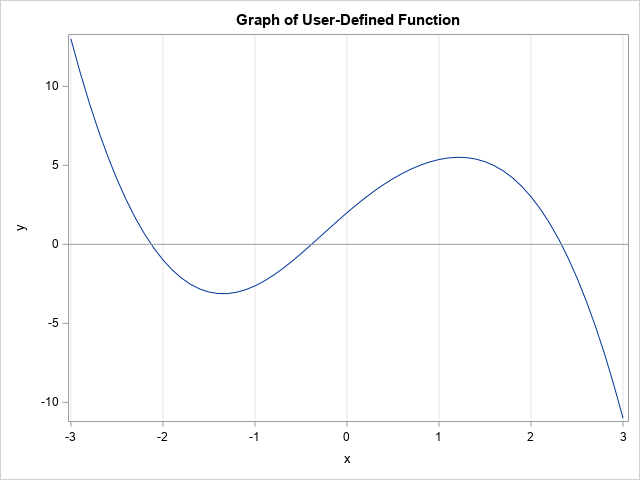From the graph, it appears that the function has three roots (zeros). The approximate locations of the roots are {-2.13, -0.38, 2.33}, as found in my previous article. The next section shows how to use the SOLVE function in PROC FCMP to find these roots.

### Use the SOLVE function to find roots

The SOLVE function is not a DATA step function. It is a "special function" in PROC FCMP. According to the documentation for the special functions, "you cannot call these functions directly from the DATA step. To use these functions in a DATA step, you must wrap the special function inside another user-defined FCMP function." You can, however, call the SOLVE function directly from procedures such as NLIN, NLMIXED, and MCMC.

I was confused when I first read the documentation for the SOLVE function, so let me give an overview of the syntax. The SOLVE function enables you to find a value x such that f(x) = y0 for a "target value", y0. Thus, the SOLVE function enables you to find roots of the function g(x) = f(x) – y0. However, in this article, I will set y0 = 0 so that x will be a root of f.

Because the function might have multiple roots, you need to provide a guess (x0) that is close to the root. The SOLVE function will start with your initial guess and apply an iterative algorithm to obtain the root. Thus, you need to provide the following information to the SOLVE function:

• The name of an FCMP function (such as "Func1") that will evaluate the function.
• An initial guess, x0, that is near a root. (You can also provide convergence criteria that tells the iterative algorithm when it has found an approximate root.)
• The parameters to the function. A missing value indicates the (one) parameter that you are solving for. For a function of one variable, you will specify a missing value for x, which tells the SOLVE function to solve for x. In general, a function can take multiple parameters, so use a missing value in the parameter list to indicate which parameter you are solving for.

The following SAS program defines a new FCMP function called Root_Func1. That function takes one argument, which is the initial guess for a root. Inside the function, the SOLVE function finds the root of the "Func1" function (defined earlier). If it was successful, it returns the root to the caller. To test the Root_Func1 function, I call it from a DATA step and pass in the values -2, 0, and +2. I obtained these guesses by looking at the graph of the Func1 function.

/* to call SOLVE in the DATA step, wrap it inside an FCMP function */ proc fcmp outlib=work.funcs.Roots; function Root_Func1(x0); array opts init absconv relconv maxiter status; /* initialize to missing */ opts = x0; /* opts is the initial guess */ z = solve("Func1", /* name of function */ opts, /* initial condition, convergence criteria, and status */ 0, /* target: find x so that f(x) = 0 */ .); /* Params for f. A missing value indicates param to solve for */ if status=0 then return( z ); /* root found */ else return (.); /* a root was not found */ endsub; quit;   data Roots1; input x0 @@; root = Root_Func1(x0); /* x0 is a "guess" for a root of Func1 */ datalines; -2 0 2 ;   proc print data=Roots1 noobs; run;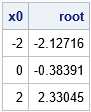The output shows that the function has found three roots, which are approximately {-2.13, -0.38, 2.33}. These are the same values that I found by using the FROOT function in SAS/IML.

### The roots of functions that have parameters

Sometimes it is useful to include parameters in a function. For example, the following function of x contains two parameters, a and b:
g(x; a, b) = exp(-x2) + a*x3 + b*x +1
Notice that the first function (Func1) is a special case of this function because f(x) = g(x; -1, 5). You can include parameters in the FCMP functions that define the function and that find the roots. When you call the SOLVE function, the last arguments are a list of parameters (x, a, b) and you should give specific values to the a and b parameters and use a missing value to indicate that the x variable is the variable that you want to solve for. This is shown in the following program:

/* you can also define a function that depends on parameters */ proc fcmp outlib=work.funcs.Roots; /* define the function. Note Func1(x) = Func2(x; a=-1, b=5) */ function Func2(x, a, b); return( exp(-x**2) + a*x**3 + b*x +1 ); endsub;   function Root_Func2(x0, a, b); array opts init absconv relconv maxiter status; /* initialize missing */ init = x0; /* pass in initial guess */ z = solve("Func2", /* name of function */ opts, /* initial condition, convergence opts, status */ 0, /* Find x so that f(x) = 0 */ ., a, b ); /* Params for f. A missing value indicates param to solve for */ if status=0 then return( z ); /* root found */ else return (.); /* a root was not found */ endsub; quit;   /* Evaluate Func2 for x in [-3,3] for three pairs of (a,b) values: (-1,5), (-1,3), and (-2,1) */ data PlotIt; do x = -3 to 3 by 0.1; y = Func2(x, -1, 5); lbl="a=-1; b=5"; output; y = Func2(x, -1, 3); lbl="a=-1; b=3"; output; y = Func2(x, -2, 1); lbl="a=-2; b=1"; output; end; run;   title "Graph of User-Defined Functions"; proc sgplot data=PlotIt; series x=x y=y / group=lbl; refline 0 / axis=y; xaxis grid; yaxis min=-10 max=10; run;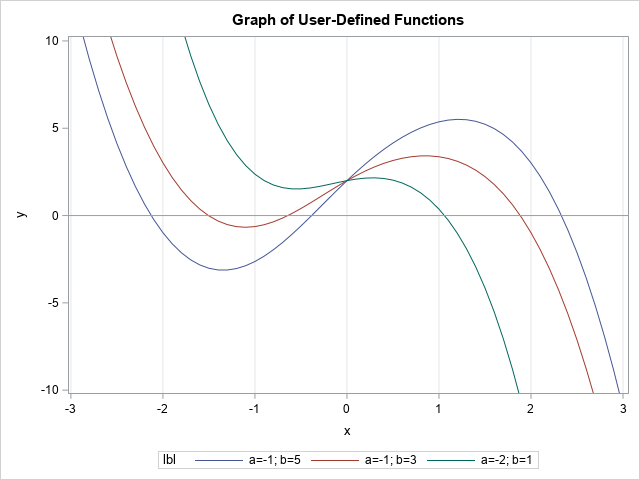The three graphs are shown. You can see that two of the graphs have three roots, but the third graph has only one root. You can call the Root_Func2 function from the DATA step to find the roots for each function:

/* find the roots of Func2 for (a,b) values (-1,5), (-1,3), and (-2,1) */ data Roots2; input a b x0; root = Root_Func2(x0, a, b); datalines; -1 5 -2 -1 5 0 -1 5 2 -1 3 -2 -1 3 0 -1 3 2 -2 1 0 -2 1 2 ;   proc print data=Roots2 noobs; run;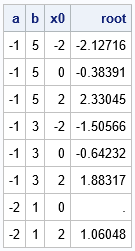Notice that the roots for (a,b) = (-1, 5) are exactly the same as the roots for Func1. The roots for the function when (a,b) = (-1, 3) are approximately {-1.51, -0.64, 1.88}. The root for the function when (a,b) = (-2, 1) is approximately 1.06.

Notice that the initial guess x0 = 0 did not converge to a root for the function that has (a,b) = (-2, 1). It is well known that Newton-type methods do not always converge. This is in contrast to Brent's methods (used by the FROOT function in SAS/IML), which always converges to a root, if one exists.

### Summary

In summary, this article shows how to use the SOLVE function in Base SAS to find the roots (zeros) of a nonlinear function of one variable. You first define a nonlinear function by using PROC FCMP. You can call the SOLVE function directly from some SAS procedures. However, if you want to use it in the DATA step, you need to define a second FCMP function that takes the function parameters and an initial guess and calls the SOLVE function. This article shows two examples of using the SOLVE function to find roots in SAS.

The post Find the root of a function by using the SAS DATA step appeared first on The DO Loop.If you have ever run a Kolmogorov-Smirnov test for normality, you have encountered the Kolmogorov D statistic. The Kolmogorov D statistic is used to assess whether a random sample was drawn from a specified distribution. Although it is frequently used to test for normality, the statistic is "distribution free" in the sense that it compares the empirical distribution to any specified distribution. I have previously written about how to interpret the Kolmogorov D statistic and how to compute it in SAS.

If you can compute a statistic, you can use simulation to approximate its sampling distribution and to approximate its critical values. Although I am a fan of simulation, in this article I show how to compute the exact distribution and critical values for Kolmogorov D statistic. The technique used in this article is from Facchinetti (2009), "A Procedure to Find Exact Critical Values of Kolmogorov-Smirnov Test."

### An overview of the Facchinetti method

Suppose you have a sample of size n and you perform a Kolmogorov-Smirnov test that compares the empirical distribution to a reference distribution. You obtain the test statistic D. To know whether you should reject the null hypothesis (that the test came from the reference distribution), you need to be able to compute the probability CDF(D) = Pr(Dn ≤ D). Facchinetti shows that you can compute the probability for any D by solving a system of linear equations. If a random sample contains n observations, you can form a certain (2n+2) x (2n+2) matrix whose entries are composed of binomial probabilities. You can use a discrete heat map to display the structure of the matrix that is used in the Facchinetti method. One example is shown below. For n=20, the matrix is 42 x 42. As Facchinetti shows in Figure 6 of her paper, the matrix has a block structure, with triangular matrices in four blocks.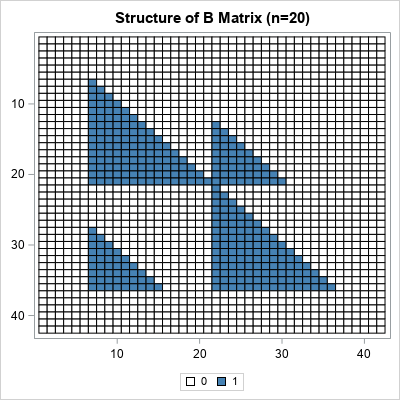The right-hand side of the linear system is also composed of binomial probabilities. The system is singular, but you can use a generalized inverse to obtain a solution. From the solution, you can compute the probability. For the details, see Facchinetti (2009).

Facchinetti includes a MATLAB program in the appendix of her paper. I converted the MATLAB program into SAS/IML and improved the efficiency by vectorizing some of the computations. You can download the SAS/IML program that computes the CDF of the Kolmogorov D statistic and all the graphs in this article. The name of the SAS/IML function is KolmogorovCDF, which requires two arguments, n (sample size) and D (value of the test statistic).

### Examples of using the CDF of the Kolmorov distribution

Suppose you have a sample of size 20. You can use a statistical table to look up the critical value of the Kolmogorov D statistic. For α = 0.05, the (two-sided) critical value for n=20 is D = 0.294. If you load and call the KolmogorovCDF function, you can confirm that the CDF for the Kolmogorov D distribution is very close to 0.95 when D=0.294:

proc iml; load module=KolmogorovCDF; /* load the function that implements the Facchinetti method */ /* simple example of calling the function */ n = 20; D = 0.29408; cdf = KolmogorovCDF(n, D); print n D cdf;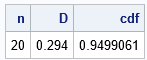The interpretation of this result is that if you draw a random samples of size 20 from the reference distribution, there is a 5% chance that the Kolmogorov D value will exceed 0.294. A test statistic that exceeds 0.294 implies that you should reject the null hypothesis at the 0.05 significance level.

### Visualize the Kolmogorov D distribution

If you can compute the CDF for one value of D, you can compute it for a sequence of D values. In this way, you can visualize the CDF curve. The following SAS/IML statements compute the CDF for the Kolmogorov D distribution when n=20 by running the computation on a sequence of D values in the open interval (0, 1):

D = do(0.01, 0.99, 0.01); /* sequence of D values */ CDF = j(1, ncol(D), .); do i = 1 to ncol(D); CDF[i] = KolmogorovCDF(n, D[i]); /* CDF for each D */ end; title "Kolmogorov CDF, n=20"; call series(D, CDF) grid={x y}; /* create a graph of the CDF */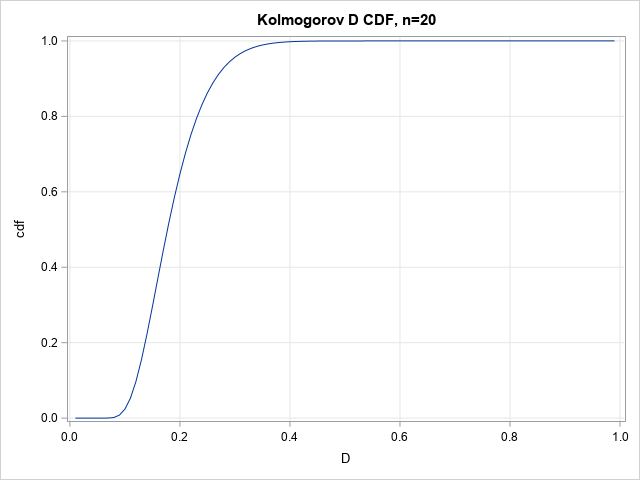The graph enables you to read probabilities and quantiles for the D20 distribution. The graph shows that almost all random samples of size 20 (drawn from the reference distribution) will have a D statistic of less than 0.4. Geometrically, the D statistic represents the largest gap between the empirical distribution and the reference distribution. A large gap indicates that the sample was probably not drawn from the reference distribution.

### Visualize a family of Kolmogorov D distributions

The previous graph was for n=20, but you can repeat the computation for other values of n to obtain a family of CDF curves for the Kolmogorov D statistic. (Facchinetti writes Dn to emphasize that the statistic depends on the sample size.) The computation is available in the program that accompanies this article. The results are shown below: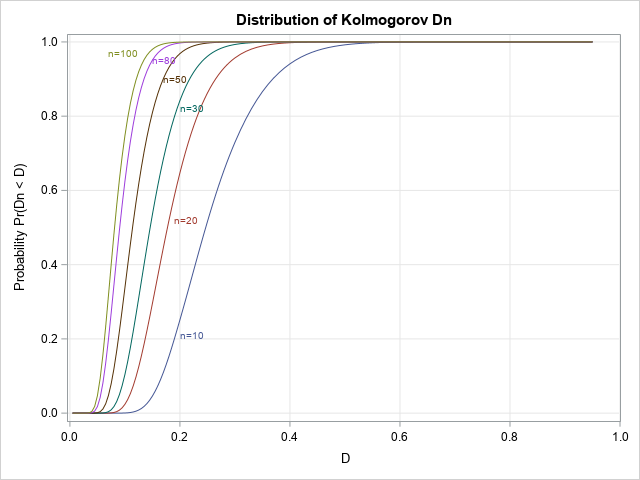This graph enables you to estimate probabilities and quantiles for several Dn distributions.

### Visualize a family of density curves

Because the PDF is the derivative of the CDF, you can use a forward difference approximation of the derivative to also plot the density of the distribution. The following density curves are derived from the previous CDF curves: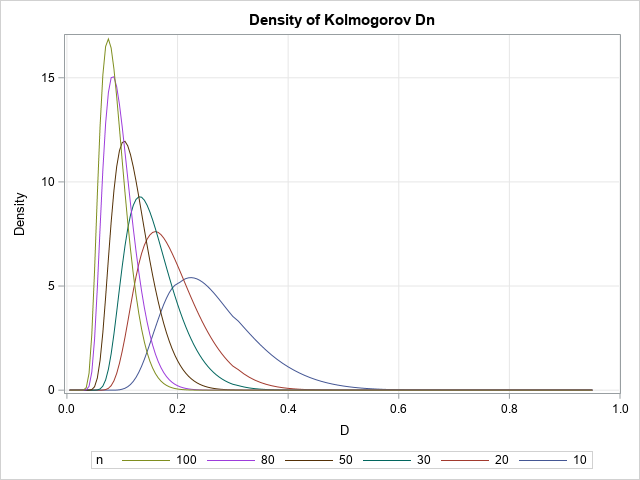### A table of critical values

If you can compute the CDF, you can compute quantiles by using the inverse CDF. You can use a root-finding technique to obtain a quantile.

In the SAS/IML language, the FROOT function can find univariate roots. The critical values of the Dn statistic are usually tabulated by listing the sample size down columns and the significance level (α) or confidence level (1-α) across the columns. Facchinetti (p. 352) includes a table of critical values in her paper. The following SAS/IML statements show how to recreate the table. For simplicity, I show only a subset of the table for n=20, 25, 20, and 35, and for four common values of α.

/* A critical value is the root of the function f(D) = KolmogorovCDF(n, D) - (1-alpha) for any specified n and alpha value. */ start KolmogorovQuantile(D) global (n, alpha); return KolmogorovCDF(n, D) - (1-alpha); finish;   /* create a table of critical values for the following vector of n and alpha values */ nVals = do(20, 35, 5); alphaVals = {0.001 0.01 0.05 0.10}; CritVal = j(ncol(nVals), ncol(alphaVals), .); do i = 1 to ncol(nVals); n = nVals[i]; do j = 1 to ncol(alphaVals); alpha = alphaVals[j]; CritVal[i,j] = froot("KolmogorovQuantile", {0.01, 0.99}); end; end; print CritVal[r=nVals c=alphaVals format=7.5 L="Critical Values (n,alpha)"];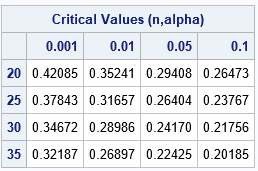For each sample size, the corresponding row of the table shows the critical values of D for which CDF(D) = 1-α. Of course, a printed table is unnecessary when you have a programmatic way to compute the quantiles.

### Summary

Facchinetti (2009) shows how to compute the CDF for the Kolmogorov D distribution for any value of n and D. The Kolmogorov D statistic is often used for goodness-of-fit tests. Facchinetti's method consists of forming and solving a system of linear equations. To make her work available to the SAS statistical programmer, I translated her MATLAB program into a SAS/IML program that computes the CDF of the Kolmogorov D distribution. This article demonstrates how to use the KolmogorovCDF in SAS/IML to compute and visualize the CDF and density of the Kolmogorov D statistic. It also shows how to use the computation in conjunction with a root-finding method to obtain quantiles and exact critical values of the Kolmogorov D distribution.

The post The Kolmogorov D distribution and exact critical values appeared first on The DO Loop.In a previous article, I discussed the definition of the Kullback-Leibler (K-L) divergence between two discrete probability distributions. For completeness, this article shows how to compute the Kullback-Leibler divergence between two continuous distributions. When f and g are discrete distributions, the K-L divergence is the sum of f(x)*log(f(x)/g(x)) over all x values for which f(x) > 0. When f and g are continuous distributions, the sum becomes an integral:
KL(f,g) = ∫ f(x)*log( f(x)/g(x) ) dx
which is equivalent to
KL(f,g) = ∫ f(x)*( log(f(x)) – log(g(x)) ) dx
The integral is over the support of f, and clearly g must be positive inside the support of f for the integral to be defined.

### The Kullback-Leibler divergence between normal distributions

I like to perform numerical integration in SAS by using the QUAD subroutine in the SAS/IML language. You specify the function that you want to integrate (the integrand) and the domain of integration and get back the integral on the domain. Use the special missing value .M to indicate "minus infinity" and the missing value .P to indicate "positive infinity."

As a first example, suppose the f(x) is the pdf of the normal distribution N(μ1, σ1) and g(x) is the pdf of the normal distribution N(μ2, σ2). From the definition, you can exactly calculate the Kullback-Leibler divergence between normal distributions:
KL between Normals: log(σ2/σ1) + (σ12 – σ22 + (μ1 – μ2)2) / (2*σ22)

You can define the integrand by using the PDF and LOGPDF functions in SAS. The following computation integrates the distributions on the infinite integral (-∞, ∞), although a "six sigma" interval about μ1, such as [-6, 6], would give the same result:

proc iml; /* Example 1: K-L divergence between two normal distributions */ start KLDivNormal(x) global(mu1, mu2, sigma1, sigma2); return pdf("Normal", x, mu1, sigma1) # (logpdf("Normal", x, mu1, sigma1) - logpdf("Normal", x, mu2, sigma2)); finish;   /* f is PDF for N(0,1) and g is PDF for N(2,3) */ mu1 = 0; sigma1 = 1; mu2 = 2; sigma2 = 3;   call quad(KL, "KLDivNormal", {.M .P}); /* integral on (-infinity, infinity) */ KLExact = log(sigma2/sigma1) + (sigma1##2 - sigma2##2+ (mu1-mu2)##2) / (2*sigma2##2); print KL KLExact (KL-KLExact)[L="Difference"];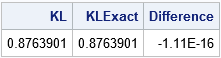The output indicates that the numerical integration agrees with the exact result to many decimal places.

### The Kullback-Leibler divergence between exponential and gamma distributions

A blog post by John D. Cook computes the K-L divergence between an exponential and a gamma(a=2) distribution. This section performs the same computation in SAS.

This is a good time to acknowledge that numerical integration can be challenging. Numerical integration routines have default settings that enable you to integrate a wide variety of functions, but sometimes you need to modify the default parameters to get a satisfactory result—especially for integration on infinite intervals. I have previously written about how to use the PEAK= option for the QUAD routine to achieve convergence in certain cases by providing additional guidance to the QUAD routine about the location and scale of the integrand. Following the advice I gave in the previous article, a value of PEAK=0.1 seems to be a good choice for the K-L divergence between the exponential and gamma(a=2) distributions: it is inside the domain of integration and is close to the mode of the integrand, which is at x=0.

/* Example 2: K-L divergence between exponential and gamma(2). Example from https://www.johndcook.com/blog/2017/11/08/why-is-kullback-leibler-divergence-not-a-distance/ */ start KLDivExpGamma(x) global(a); return pdf("Exponential", x) # (logpdf("Exponential", x) - logpdf("Gamma", x, a)); finish;   a = 2; /* Use PEAK= option: https://blogs.sas.com/content/iml/2014/08/13/peak-option-in-quad.html */ call quad(KL2, "KLDivExpGamma", {0 .P}) peak=0.1; print KL2;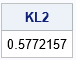The value of the K-L divergence agrees with the answer in John D. Cook's blog post.

### Approximating the Exponential Distribution by the Gamma Distribution

Recall that the K-L divergence is a measure of the dissimilarity between two distributions. For example, the previous example indicates how well the gamma(a=2) distribution approximates the exponential distribution. As I showed for discrete distributions, you can use the K-L divergence to select the parameters for a distribution that make it the most similar to another distribution. You can do this by using optimization methods, but I will merely plot the K-L divergence as a function of the shape parameter (a) to indicate how the K-L divergence depends on the parameter.

You might recall that the gamma distribution includes the exponential distribution as a special case. When the shape parameter a=1, the gamma(1) distribution is an exponential distribution. Therefore, the K-L divergence between the exponential and the gamma(1) distribution should be zero.

The following program computes the K-L divergence for values of a in the range [0.5, 2] and plots the K-L divergence versus the parameter:

/* Plot KL(Expo, Gamma(a)) for various values of a. Note that gamma(a=1) is the exponential distribution. */ aa = do(0.5, 2, 0.01); KL = j(1, ncol(aa), .); do i = 1 to ncol(aa); a = aa[i]; call quad(KL_i, "KLDivExpGamma", {0 .P}) peak=0.1; KL[i] = KL_i; end; title "Kullback-Leibler Divergence Between Exponential and Gamma{a)"; call series(aa, KL) grid={x y} label={'Gamma Shape Parameter (a)' 'K-L Divergence'};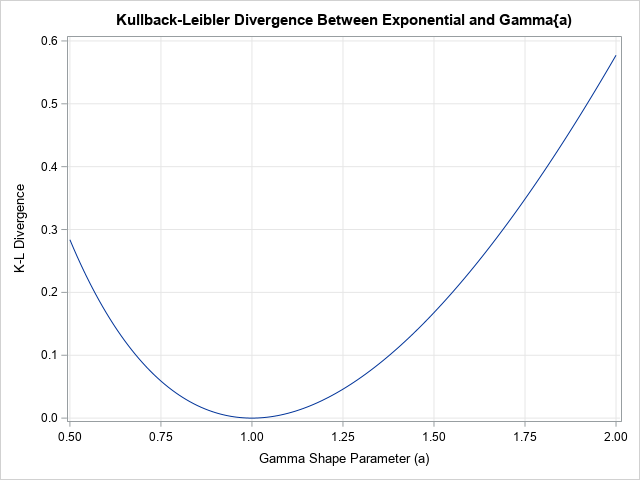As expected, the graph of the K-L divergence reaches a minimum value at a=1, which is the best approximation to an exponential distribution by the gamma(a) distribution. Note that the K-L divergence equals zero when a=1, which indicates that the distributions are identical when a=1.

### The Kullback-Leibler divergence between two continuous probability distributions is an integral. This article shows how to use the QUAD function in SAS/IML to compute the K-L divergence between two probability distributions.

The post The Kullback–Leibler divergence between continuous probability distributions appeared first on The DO Loop.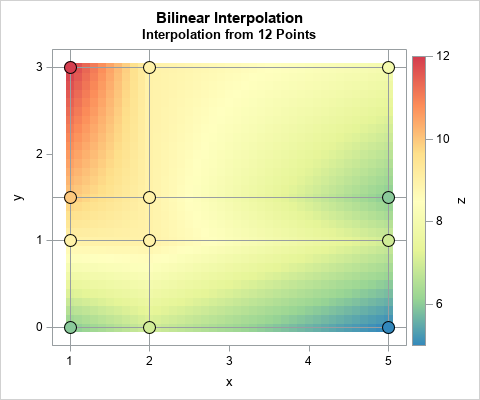This article shows how to perform two-dimensional bilinear interpolation in SAS by using a SAS/IML function. It is assumed that you have observed the values of a response variable on a regular grid of locations.

A previous article showed how to interpolate inside one rectangular cell. When you have a grid of cells and a point (x, y) at which to interpolate, the first step is to efficiently locate which cell contains (x, y). You can then use the values at the corners of the cell to interpolate at (x, y), as shown in the previous article. For example, the adjacent graph shows the bilinear interpolation function that is defined by 12 points on a 4 x 3 grid.

### Bilinear interpolation assumptions

For two-dimensional linear interpolation of points, the following sets of numbers are the inputs for bilinear interpolation:

1. Grid locations: Let x1 < x2 < ... < xp be the horizontal coordinates of a regular grid. Let y1 < y2 < ... < yn be the vertical coordinates.
2. Response Values: Let Z be an n x p matrix of response values on the grid. The (j, i)th value of Z is the response value at the location (xi, yj). Notice that the columns of Z correspond to the horizontal positions for the X values and the rows of Z correspond to the vertical positions for the Y values. (This might seem "backwards" to you.) The response values data should not contain any missing values. The X, Y, and Z values (called the "fitting data") define the bilinear interpolation function on the rectangle [x1, xp] x [y1, yn].
3. Values to score: Let (s1, t1), (s2, t2), ..., (sk, tk) be a set of k new (x, y) values at which you want to interpolate. All values must be within the range of the data: x1 ≤ si ≤ xp and y1 ≤ si ≤ yn for all (i,j) pairs. These values are called the "scoring data" or the "query data."

The goal of interpolation is to estimate the response at each location (si, tj) based on the values at the corner of the cell that contains the location. I have written a SAS/IML function called BilinearInterp, which you can freely download and use. The following statements indicate how to define and store the bilinearInterp function:

proc iml; /* xGrd : vector of Nx points along x axis yGrd : vector of Ny points along y axis z : Ny x Nx matrix. The value Z[i,j] is the value at xGrd[j] and yGrd[i] t : k x 2 matrix of points at which to interpolate The function returns a k x 1 vector, which is the bilinear interpolation at each row of t. */ start bilinearInterp(xgrd, ygrd, z, t); /* download function from https://github.com/sascommunities/the-do-loop-blog/blob/master/interpolation/bilinearInterp.sas */ finish; store module=bilinearInterp; /* store the module so it can be loaded later */ QUIT;

### The structure of the fitting data

The fitting data for bilinear interpolation consists of the grid of points (Z) and the X and Y locations of each grid point. The X and Y values do not need to be evenly spaced, but they can be.

Often the fitting data are stored in "long form" as triplets of (X, Y, Z) values. If so, the first step is to read the triplets and convert them into a matrix of Z values where the columns are associated with the X values and the rows are associated with the Y values. For example, the following example data set specifies a response variable (Z) on a 4 x 3 grid. The values of the grid in the Y direction are {0, 1, 1.5, 3} and the values in the X direction are {1, 2, 5}.

As explained in the previous article, the data must be sorted by Y and then by X. The following statements read the data into a SAS/IML matrix and extract the grid points in the X and Y direction:

data Have; input x y z; datalines; 1 0 6 2 0 7 5 0 5 1 1 9 2 1 9 5 1 7 1 1.5 10 2 1.5 9 5 1.5 6 1 3 12 2 3 9 5 3 8 ;   /* If the data are not already sorted, sort by Y and X */ proc sort data=Have; by Y X; run;   proc iml; use Have; read all var {x y z}; close; xGrd = unique(x); /* find grid points */ yGrd = unique(y); z = shape(z, ncol(yGrd), ncol(xGrd)); /* data must be sorted by y, then x */ print z[r=(char(yGrd)) c=(char(xGrd))];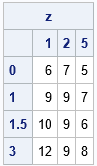Again, note that the columns of Z represent the X values and the rows represent the Y values. The xGrd and yGrd vectors contain the grid points along the horizontal and vertical dimensions of the grid, respectively.

### The structure of the scoring data

The bilinearInterp function assumes that the points at which to interpolate are stored in a k x 2 matrix. Each row is an (x,y) location at which to interpolate from the fitting data. If an (x,y) location is outside of the fitting data, then the bilinearInterp function returns a missing value. The scoring locations do not need to be sorted.

The following example specifies six valid points and two invalid interpolation points:

t = {0 0, /* not in data range */ 1 1, 1 2, 2.5 2, 4 0.5, 4 2, 5 3, 6 3}; /* not in data range */   /* LOAD the previously stored function */ load module=bilinearInterp; F = bilinearInterp(xGrd, yGrd, z, t); print t[c={'x' 'y'}] F[format=Best4.];The grid is defined on the rectangle [1,5] x [0,3]. The first and last rows of t are outside of the rectangle, so the interpolation returns missing values at those locations. The locations (1,1) and (5,3) are grid locations (corners of a cell), and the interpolation returns the correct response values. The other locations are in one of the grid cells. The interpolation is found by scaling the appropriate rectangle onto the unit square and applying the bilinear interpolation formula, as shown in the previous article.

### Visualizing the bilinear interpolation function

You can use the ExpandGrid function to generate a fine grid of locations at which to interpolate. You can visualize the bilinear interpolation function by using a heat map or by using a surface plot.

The heat map visualization is shown at the top of this article. The colors indicate the interpolated values of the response variable. The markers indicate the observed values of the response variable. The reference lines show the grid that is defined by the fitting data.

The following graph visualizes the interpolation function by using a surface plot. The function is piecewise quadratic. Within each cell, it is linear on lines of constant X and constant Y. The "ridge lines" on the surface correspond to the locations where two adjacent grid cells meet.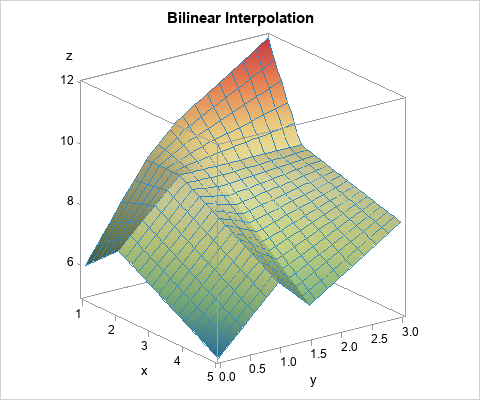### Summary

In summary, you can perform bilinear interpolation in SAS by using a SAS/IML function. The function uses fitting data (X, Y, and Z locations on a grid) to interpolate. Inside each cell, the interpolation is quadratic and is linear on lines of constant X and constant Y. For each location point, the interpolation function first determines what cell the point is in. It then uses the corners of the cell to interpolate at the point. You can download the SAS program that performs bilinear interpolation and generates the tables and graphs in this article.

The post Bilinear interpolation in SAS appeared first on The DO Loop.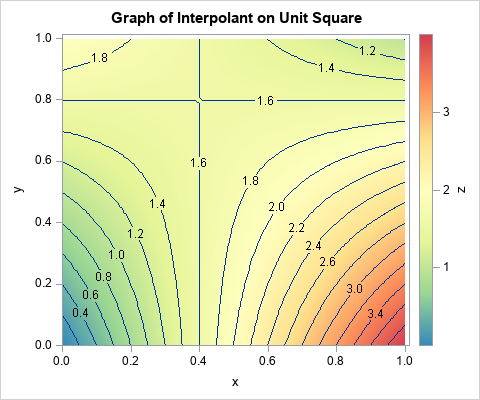I've previously written about linear interpolation in one dimension. Bilinear interpolation is a method for two-dimensional interpolation on a rectangle. If the value of a function is known at the four corners of a rectangle, an interpolation scheme gives you a way to estimate the function at any point in the rectangle's interior. Bilinear interpolation is a weighted average of the values at the four corners of the rectangle. For an (x,y) position inside the rectangle, the weights are determined by the distance between the point and the corners. Corners that are closer to the point get more weight. The generic result is a saddle-shaped quadratic function, as shown in the contour plot to the right.

The Wikipedia article on bilinear interpolation provides a lot of formulas, but the article is needlessly complicated. The only important formula is how to interpolate on the unit square [0,1] x [0,1]. To interpolate on any other rectangle, simply map your rectangle onto the unit square and do the interpolation there. This trick works for bilinear interpolation because the weighted average depends only on the relative position of a point and the corners of the rectangle. Given a rectangle with lower-left corner (x0, y0) and upper right corner (x1, y1), you can map it into the unit square by using the transformation (x, y) → (u, v) where u = (x-x0)/(x1-x0) and v = (y-y0)/(y1-y0).

### Bilinear interpolation on the unit square

Suppose that you want to interpolate on the unit square. Assume you know the values of a continuous function at the corners of the square:

• z00 is the function value at (0,0), the lower left corner
• z10 is the function value at (1,0), the lower right corner
• z01 is the function value at (0,1), the upper left corner
• z11 is the function value at (1,1), the upper right corner

If (x,y) is any point inside the unit square, the interpolation at that point is the following weighted average of the values at the four corners:
F(x,y) = z00*(1-x)*(1-y) + z10*x*(1-y) + z01*(1-x)*y + z11*x*y

Notice that the interpolant is linear with respect to the values of the corners of the square. It is quadratic with respect to the location of the interpolation point. The interpolant is linear along every horizontal line (lines of constant y) and along every vertical line (lines of constant x).

### Implement bilinear interpolation on the unit square in SAS

Suppose that the function values at the corners of a unit square are z00 = 0, z10 = 4, z01 = 2, and z11 = 1. For these values, the bilinear interpolant on the unit square is shown at the top of this article. Notice that the value of the interpolant at the corners agrees with the data values. Notice also that the interpolant is linear along each edge of the square. It is not easy to see that the interpolant is linear along each horizontal and vertical line, but that is true.

In SAS, you can use the SAS/IML matrix language to define a function that performs bilinear interpolation on the unit square. The function takes two arguments:

• z is a four-element that specifies the values of the data at the corners of the square. The values are specified in the following order: lower left, lower right, upper left, and upper right. This is the "fitting data."
• t is a k x 2 matrix, where each row specifies a point at which to interpolate. This is the "scoring data."

In a matrix language such as SAS/IML, the function can be written in vectorized form without using any loops:

proc iml; start bilinearInterpSquare(z, t); z00 = z; z10 = z; z01 = z; z11 = z; x = t[,1]; y = t[,2]; cx = 1-x; /* cx = complement of x */ return( (z00*cx + z10*x)#(1-y) + (z01*cx + z11*x)#y ); finish;   /* specify the fitting data */ z = {0 4, /* bottom: values at (0,0) and (1,0) */ 2 1}; /* top: values at (0,1) and (1,1) */ print z[c={'x=0' 'x=1'} r={'y=0' 'y=1'}];   t = {0.5 0, /* specify the scoring data */ 0 0.25, 1 0.66666666, 0.5 0.75, 0.75 0.5 }; F = bilinearInterpSquare(z, t); /* test the bilinear interpolation function */ print t[c={'x' 'y'} format=FRACT.] F;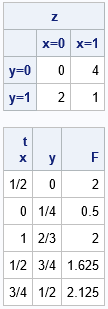For points on the edge of the square, you can check a few values in your head:

• The point (1/2, 0) is halfway between the corners with values 0 and 4. Therefore the interpolation gives 2.
• The point (0, 1/4) is a quarter of the way between the corners with values 0 and 2. Therefore the interpolation gives 0.5.
• The point (1, 2/3) is two thirds of the way between the corners with values 4 and 1. Therefore the interpolation gives 2.

The other points are in the interior of the square. The interpolant at those points is a weighted average of the corner values.

### Visualize the interpolant function

Let's use the bilinearInterpSquare function to evaluate a grid of points. You can use the ExpandGrid function in SAS/IML to generate a grid of points. When we look at the values of the interpolant on a grid or in a heat map, we want the X values to change for each column and the Y values to change for each row. This means that you should reverse the usual order of the arguments to the ExpandGrid function:

/* for visualization, reverse the arguments to ExpandGrid and then swap 1st and 2nd columns of t */ xGrd = do(0, 1, 0.2); yGrd = do(0, 1, 0.2); t = ExpandGrid(yGrd, xGrd); /* want X changing fastest; put in 2nd col */ t = t[ ,{2 1}]; /* reverse the columns from (y,x) to (x,y) */ F = bilinearInterpSquare(z, t); Q = shape(F, ncol(yGrd), ncol(xGrd)); print Q[r=(char(YGrd,3)) c=(char(xGrd,3)) label="Bilinear Interpolant"];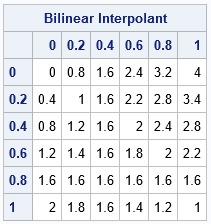The table shows the interpolant evaluated at the coordinates of a regular 6 x 6 grid. The column headers give the coordinate of the X variable and the row headers give the coordinates of the Y variable. You can see that each column and each row is an arithmetic sequence, which shows that the interpolant is linear on vertical and horizontal lines.

You can use a finer grid and a heat map to visualize the interpolant as a surface. Notice that in the previous table, the Y values increase as you go down the rows. If you want the Y axis to point up instead of down, you can reverse the rows of the grid (and the labels) before you create a heat map:

/* increase the grid resolution and visualize by using a heat map */ xGrd = do(0, 1, 0.1); yGrd = do(0, 1, 0.1); t = ExpandGrid(yGrd, xGrd); /* want X changing fastest; put in 2nd col */ t = t[ ,{2 1}]; /* reverse the columns from (y,x) to (x,y) */ F = bilinearInterpSquare(z, t); Q = shape(F, ncol(yGrd), ncol(xGrd));   /* currently, the Y axis is pointing down. Flip it and the labels. */ H = Q[ nrow(Q):1, ]; reverseY = yGrd[ ,ncol(yGrd):1]; call heatmapcont(H) range={0 4} displayoutlines=0 xValues=xGrd yValues=reverseY colorramp=palette("Spectral", 7)[,7:1] title="Interpolation at Multiple Points in the Unit Square";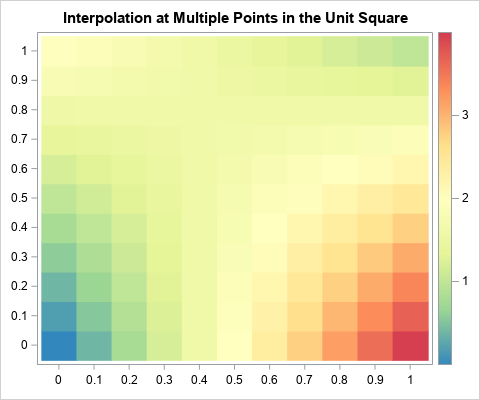The heat map agrees with the contour plot at the top of this article. The heat map also makes it easier to see that the bilinear interpolant is linear on each row and column.

In summary, you can create a short SAS/IML function that performs bilinear interpolation on the unit square. (You can download the SAS program that generates the tables and graphs in this article.) The interpolant is a quadratic saddle-shaped function inside the square. You can use the ideas in this article to create a function that performs bilinear interpolation on an arbitrary grid of fitting data. The next article shows a general function for bilinear interpolation in SAS.

The post What is bilinear interpolation? appeared first on The DO Loop.